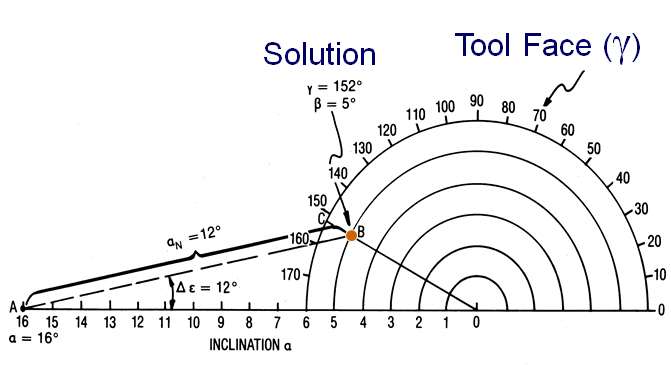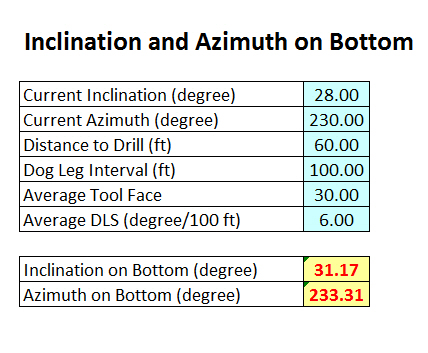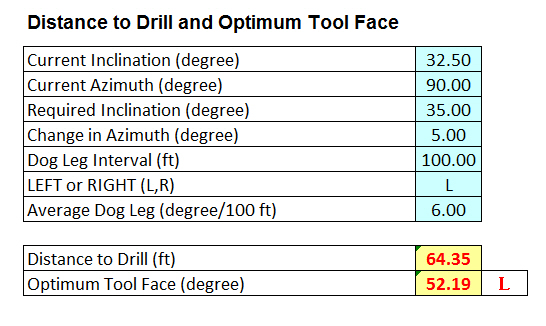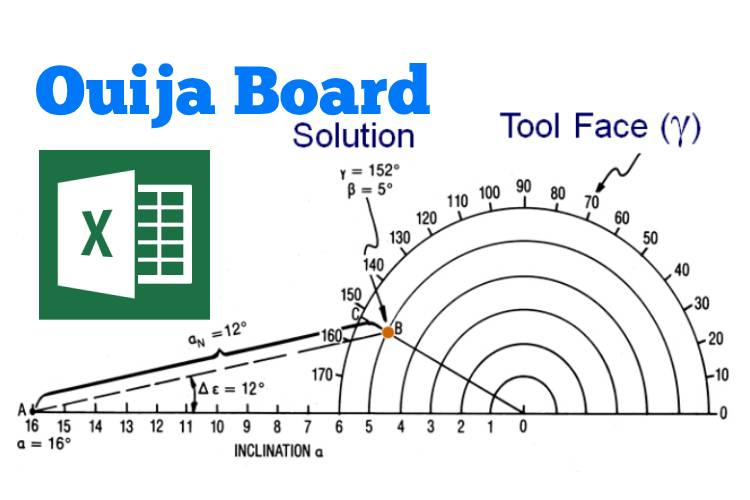# Ouija Board Directional Drilling Excel Spreadsheet

Ouija Board is a method that directional drillers use to calculate inclination and azimuth at the bottom or distance and tool face to drill at required direction. Figure 1 is an Ouija board.Figure 1 – Ouija Board

We got an excellent Excel Spreadsheet that will help you calculate the direction at bottom, distance to drill and tool face. We will give you a couple of examples to give you an idea what results from the Ouija Board spread sheet.

These are current directional parameters

Current Inclination (degree) = 28.00

Current Azimuth (degree) = 230.00

Distance to Drill (ft) = 60.00

Dog Leg Interval (ft) = 100.00

Average Tool Face = 30.00

Average DLS (degree/100 ft) = 6.00

Result is “Inclination and Azimuth on Bottom”.

Inclination on Bottom (degree) = 31.17

Azimuth on Bottom (degree) = 233.31

You can use this part to project the inclination and azimuth at the bottom.Figure 2 – Inclination and Azimuth on Bottom

This is another example below.

Current Inclination (degree) = 32.50

Current Azimuth (degree) = 90.00

Required Inclination (degree) = 35.00

Change in Azimuth (degree) = 5.00

Dog Leg Interval (ft) = 100.00

LEFT or RIGHT (L,R) = L

Average Dog Leg (degree/100 ft) = 6.00

Result is “Distance to Drill and Optimum Tool Face”.

Distance to Drill (ft) = 64.35

Optimum Tool Face (degree) = 52.19 LFigure 3 – Distance to Drill and Optimum Tool Facehttps://oilfieldmania.files.wordpress.com/2015/07/ouija-board-final.xlsx

Share the joy
Tagged , . Bookmark the permalink.1.jason joos says:
•DrillingFormulas.Com says: